## Important Statistical Distributions For Oracle Database Tuning...Really

Posted on 02-Feb-2011 by Craig Shallahamer, craig@orapub.com

These past two months I have been doing a tremendous amount of Oracle Database performance tuning research work. (I love it and it's being poured into my training courses.) I have been driven to achieve a deeper understanding of the statistic average and what it implies and what is it worth in Oracle Database performance tuning. As you will soon see in subsequent posts, understanding statistical distributions has been absolutely key to carrying out the research. I also plan to reference this posting in future postings.

Please, if you have run away from statistics before, I promise this will be different. It's written for the typical Oracle DBA, which means I wrote it for you and therefore I'm really hoping it makes lots of sense and will be useful in your career. So please...read on.

### My Approach

My approach is to start with introducing the histogram. I'm doing this because it is a wonderful visual way to compare and contrast experimental sample sets and of course, statistical distributions. Then I will move on and introduce five different distributions. I didn't just randomly pick five. I chose these five because they are important for our work. For each distribution I start by presenting an simple non-Oracle example. Then I get a little more technical by describing the inputs to create the distribution, for example the average. I also include other interesting tidbits. Here we go...

### Understanding a Histogram

When presented with a sample set, such as {1.5, 3.2, 2.6, 4.2, 3.8, 2.1 , 5.1, 2.6, 6.5, 3.4, 4.2}, a fantastic way to visually grasp the data and to get a gut feel about it, is to create a histogram. The image below was created by simply copying and pasting the previous sample set directly into WolframAlpha...give it a try.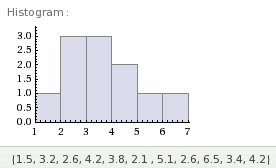A histogram's vertical axis is the number of occurrences which, in our case is the number of samples. In our sample set there are 11 samples, which means there are 11 occurrences. Each of these 11 occurrences will be represented somewhere in the histogram.

The horizontal axis are the values of the samples. Scanning our 11 sample values you'll notice the minimum value is 1.5 and the maximum value is 6.5. The horizontal axis must include this range. For our sample set's histogram, the minimum value on the histogram is 1 and the maximum value is 7.

Our first sample is 1.5 and is represented on this histogram as the bottom (that is, first) occurrence on the first bar from the left. The second sample 3.2 is represented as the first occurrence on the third bar from the left. Notice there are three samples between 3 and 4, hence that histogram bar is 3 occurrences high. If you count the number of occurrences in the histogram, you'll notice there are 11, which is also the number of our samples!

What is interesting in this sample set's histogram is we can see the distribution is skewed to the left with a somewhat long right tail. The mean, that is the average, is 3.6 and the median is 3.4. This tells us there are more samples to left of the mean than to right of the mean. Recognizing this difference is very important in Oracle performance analysis.

That's the end of my introduction to histograms. The key is every sample is represented somewhere on the graph. Next I'm going to introduce the five statistical distributions every Oracle performance analyst needs to know about; uniform, normal, exponential, poisson, and finally the log normal distribution.

### The Uniform Distribution

Every programmer at some point has needed a random number. Most random number generators provide the ability to return a random number between two values, say 0 and 100. There is an important underlying assumption we typically don't think about. This assumption is any number is just as likely to be returned as any other number. Said another way, the likelihood of returning a 15 is just as likely as returning 55. Said yet another way, there is no preference toward returning a specific number or a group of numbers. This is another way of saying the distribution of results is uniform...hence the uniform distribution.

Let's start with a specific quantify and numeric range of random numbers and place them into a histogram. The histogram below is based on a set of only 10 random real numbers between 0 and 100. I also specified there to be 10 histogram bins, that is, groups or buckets. I defined the random set of values by providing the minimum and maximum values.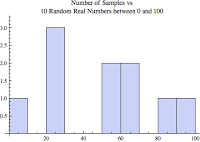By the way, in the figure above you should be able to count the total number of samples which, should add up to the number of samples (10). That doesn't look very random because it contains only 10 values. If I increase the number of samples from 10 to 10000 the histogram looks very different. Below is the histogram containing 10000 random real numbers between 0 and 100.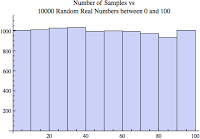This is more like it and what we expected to see. A way of interpreting this histogram is that we are about as likely to pick any number between 0 and 100... it's like random! ...that's because the sample set is full of random uniformly distributed numbers.

The median for a uniform distribution is the same as the mean. If you were to sort all the samples and pick the one in the middle that would be the median and also the mean.

### The Normal Distribution

If I asked a group of people to measure the length of a physically present piece of wood down to the millimeter, I will receive a variety of answers. If took all the results (my sample set) and placed them into a histogram format, the result would be the classic bell curve, which is more formally known as the normal distribution.

The key thing to remember about a normal distribution is there are just as many samples less than the mean than there are greater than the mean. This is another way of saying the median is equal to the mean.

A normal distribution set of values is defined by its mean and standard deviation (which is a statistic that tell us about the dispersion of the samples). If I had a really cool random number generator, I could tell it to return a set of numbers that are normally distributed, with a mean of m, and a standard deviation of s. Thankfully, I do have a spiffy random number generator like this! I used a Mathematica command

(actually called a symbol, not a command) to generate a sample set used to create the below histograms!

The histogram below is based on a set of only 10 normally distributed real numbers with a mean of 50 and a standard deviation of 2. I also specified there to be 10 bins, that is, groups or buckets.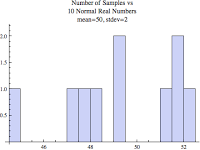That doesn't look very normal! That's because there are only 10 samples, but if we increase the number of samples to 10000 it looks more, well...normal.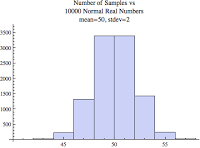But still, it's not that smooth because I set the number of bins to 10. If I let Mathematica automatically set the number of bins, we see the classic looking normal distribution histogram. Remember these three "normal" distribution images have the same mean (average) and standard deviation.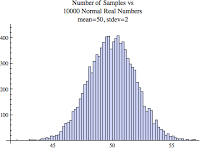The median for a normal distribution is the same as the mean. If you where to sort all the samples and pick the one in the middle that would be both the median and also the mean.

The normal distribution is often used because it makes things easy, most people know what the histogram looks like, the math is more straightforward, and students are used to working with normally distributed data. People are drawn to symmetry...we want symmetry. If we see something that is not symmetrical, it's like we are forced to understand why...an that takes energy and time. So most people, including research scientists, tend to assume their data is normal. [Log-normal Distributions across the Science: Key and Clues, BioScience, May 2001] In my Forecasting Oracle Performance book

, you will notice I write many times,"...assuming the samples are normally distributed..." By stating this, I am saying the math I'm going to use will work well only when the sample set is normally distributed. If the distribution is not normal, while the math will crank out a result, it will not be as reliable.

As you dig deeper into Oracle performance analysis, you'll begin to realize that many performance related distributions are indeed not normal. Most people think that most distributions are normal, but as I'm demonstrating in my blog entries there are many situations where this is not true...especially when we're talking about Oracle performance topics.

### The Exponential Distribution

Radioactive material decays exponentially. For example, suppose in the next hour a piece of radioactive material has a 10% chance of splitting, therefore having a 90% chance of not splitting. Because radioactive decay occurs exponentially, within the next hour there will be a 5% chance the material will split and a 95% chance it will not split. Within the third hour there will be a 2.5% chance the material will split, with a 97.5% chance it will not split. And on and on...unfortunately forever. Here is our sample set in WolframAlpha-ready

format, followed by the plot:

``````
plot {{1,5},{2,2.5},{3,1.25},{4,0.625},{5,0.313},{6,0.156},{7,0.078}} ``````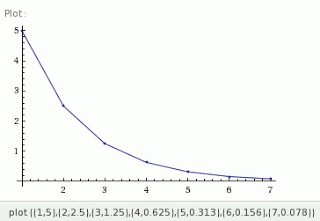Crack open a book about queuing theory or computing system performance analysis and you'll see the words exponential distribution. It's one of the phrases that computer folks throw around, only few can talk about, and very few really understand. So in a few short paragraphs, I'm going to try and explain this (that's the "talk about" part) as clearly as I possibly can, without the aid of a white board and personal classroom interaction. If you want personal classroom interaction check out my courses.

The exponential distribution is like most other distributions in that it is defined by a small set of parameters. For the uniform distribution, the sample set definition is x number of random numbers (i.e., integers, real, etc.) between a minimum and maximum value. For the normal distribution, the sample set definition is x number of normally distributed numbers (i.e., integers, real, etc.) having a mean of m and a standard deviation of s. For the exponential distribution, the sample set definition is x number of exponentially distributed numbers having an average of m. (Actually the real input parameter is 1/m). There is only a single parameter!

Unlike a uniform or normal distribution, there are more samples less than the mean then greater than the mean! If you understand the second point above, then it will make sense that if you where to sort all the samples and pick the one in the middle (the median sample) its value would be less than the mean. Said another way, there are more samples less than the mean then greater then the mean. You can see this visually in the figures below. In the radioactive decay example above, the mean is 1.42 and the median is 0.625.

Exponentially distributed sample sets are very common in computing system performance analysis. It is a given in capacity planning that the time between transaction arrivals (one of my next blog entries) and also how long it takes to service the transaction (not their waiting or queue time but the actual service) is exponentially distributed.

The histogram below is based on a set of only 10 exponentially distributed real numbers with a mean of 50. I also specified there to be 10 bins, that is, groups or buckets.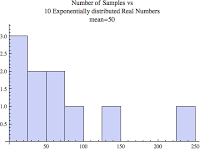Because there are only 10 samples, the histogram is very awkward looking. But even with only 10 samples, it seems to look different then both the 10 sample uniform and normal distribution histograms shown above. This histogram is also eerily similar to the histogram near the top of this blog based on the 12 sample SQL execution elapsed times...woops... sorry...that's at the top of my next blog posting!

The below two figures are based on the exact same sample set. The only difference is how I defined the histogram bins.

The figure below is still an exponentially distributed set of values with a mean of 50 but I increased the number of samples from only 10 to 10000.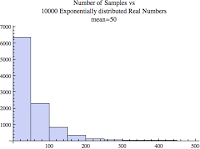It is still chunky looking but that's because I set the number of bins to 10. I wanted to show you this because many times when doing experiments and performance analysis we don't have 10000 samples and so there may only be a few bins. Even with only 10 bins, the above pattern or look is what you might see. Letting Mathematica set the number of bins, we get the classic looking exponential distribution histogram.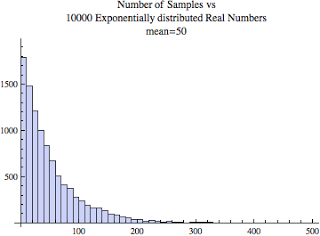Remember, all three of the above histograms has a mean of 50. The only difference is the number of samples and the number of histogram bins.

Remember, the above two images/histograms are based on the exact same sample set and the only difference in the bin size settings!

Let's investigate the median a bit. As you'll recall if we sorted all the samples and picked the middle sample, that will be the median. Looking at the directly above 10000 sample histogram above, what does the mean and the median look to be? Well, I told you the mean is 50, so what about the median? Because the median is based on the number of samples not their values, in this case the median will be less than the mean. It's like those few large values have pulled the mean to right and away from the median. While you can't see this in the above histogram graphic, there are samples with values of 300 to 400 to 500. There are not a lot of them, but they are there and they are effectively pulling the mean toward them!

While the mean for the above histogram is 50, the median is about 35. So our hunch is correct in that the median is less than the mean.

While many Oracle performance distributions may look exponential, if we increase the bin size they begin to look poisson-like. So it's important we also investigate the poisson distribution.

### Poisson Distribution

Suppose I'm doing a traffic study and need to estimate the average number of vehicles that pass by a specific free-flowing(1) destination each minute. I wake up one delightful morning, get my cup of coffee, arrive at my destination and start counting. Every 30 seconds we record the number of vehicles that pass by. Let's say I did this for 10 minutes, which means I would have 20 samples. The result sample set is poisson distributed. Assuming I actually did this, here are the actual 20 sample values, followed by the histogram.

``{19, 19, 18, 10, 20, 16, 12, 18, 15, 15, 18, 18, 16, 20, 17, 14, 27, 19, 14, 17}  ``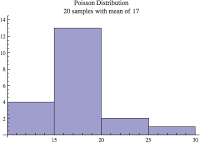You can see a similar histogram by copying and pasting the above values (keep the curly braces) into WolframAlpha.

(1) By free-flowing I mean each vehicle that passes is not somehow dependent on another vehicle that passes. For example, if there is a traffic jam, an accident, or a stop sign nearby causing the vehicles to bunch up, then the vehicle arrivals would be related. This is another way of saying the arrivals must be independent which, must occur in a true poisson distribution. Additionally, the inter-arrival times (i.e., time between each arrival) must be exponentially distributed.

The poisson histogram image above is very typical. Going left to right, there is a quick build up to the peak and then then a slow build down. If we have enough samples, like the normal distribution, the mean will equal the median. So even though there are a few large values to the right of the mean, there are enough smaller values to the left of the mean to keep the mean and median "in check" and not swaying from each other. As you'll recall from above, this is very different compared to an exponential distribution. With an exponential distribution the far left histogram bar is the tallest, that is it contains the most samples, and hence there is not built up.

Take a look at the below two histograms. Are they exponential or poisson? It is natural to think the distribution on the left is exponential and the distribution on the right is poisson... But actually, they are both poisson.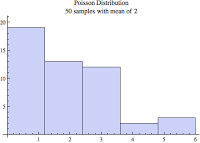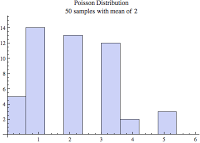When comparing the above two images, the image on the left (contains the wider bins) looks more exponential than poisson. Surprisingly, they are both based on the exact same sample set! If you look closely, you'll notice the only difference is the histogram bin size. You can also be deceived when there are lots of samples because a poisson distribution can look like a normal distribution! Just look at the image below.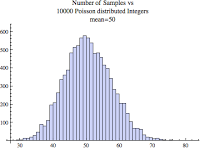I will get more into a poisson process and its distribution in my upcoming blog entry on SQL statement arrival rates. But for now, keep in mind that what may appear to be a normal or exponential distribution could actually be more poisson-like! They can be difficult to distinguish. The only way to test this is to perform a statistical hypothesis test.

As I will blog about soon, many Oracle performance distribution may look exponential or poisson, but they fail a statistical hypothesis test. There is yet another and lesser known distribution that many times is the best match for Oracle performance related sample sets. It's called the log normal distribution.

### Log Normal Distribution

There are times when a skewed normal distribution occurs. By skew I mean the tallest histogram bar is not in the middle but to the left or the right. For example, a skew is likely when mean values are near zero, variances are large and perhaps extreme, and the sample values cannot be negative. When these types of conditions exist, the log normal distribution may best describe the sample set.

Personal income, reaction time to snake bites and bee stings, and country GDP and oil field reserves are all supposed to be log normal distributed. Humm...

Proving it to myself

To prove this to myself, I decided to give this a try using real country GDP and country oil reserve data. With my hands shaking in anticipation, I called once again on Mathematica. Mathematica has vast data resources that can be pulled onto my desktop and analyzed. It's scary-amazing. Within minutes I had the GDP and oil reserves for 231 countries at my fingertips. I'm not sure of the year, but that's really not important anyways.

I then placed the data into a histogram. Regardless of my histogram tweaks, the image always looked exponentially distributed. However, when I performed a statistical fitness tests comparing the data samples with the distributions presented in this blog, only the log normal distribution was statistically similar. Every other distribution did not conform to the actual data. So I guess the experts where correct.

How to create a log normal data set

First, I'll state a simple definition: A sample set is log-nomal if log(sample x) is normally distributed. For example, if I have 100 log-normal samples and I apply log(sample[i]) to each one and then create a histogram from the results, the histogram will be normally distributed!

Here is how I created a log normal sample set taking a slightly different twist: For each sample x in a normal distribution with a given mean and standard deviation, apply exp(x) to it and place the result into another sample set. If you create a histogram on the new exp(x) samples it will look this like this (assuming the normal distribution samples have a mean of 3 and the standard deviation of 1.5):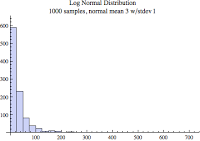It looks a lot like the exponential and poisson distributions! In fact, based on it's two input parameters (mean and standard deviation from its associated normal distribution), it can look like either one...especially if we mess with the histogram bin sizes and number. I suspect this flexibility is what makes it a relatively good visual match for our experimental data.

Now this is really cool! Recall the country GDP sample set I mentioned just above in the Proving it to myself section. Below is the country GDP histogram, with 80 bins and showing 90% of the data.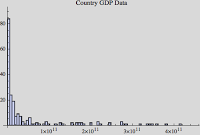If I take the log of each country's GDP and create a histogram of the result, it looks like this: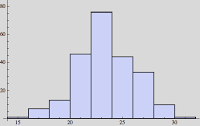Very cool, eh? So while the raw data histogram looks more exponential, when we apply the log function to the data and create a histogram it looks pretty normal...which means visually our country GDP data is log normal distributed. And as I mentioned above, performing a statistical hyposthesis test the data is statistically log normal as well.

### How to lie using histograms

Interestingly, the image below is based on the exact same sample set as the Figure X histogram three images above. The only difference is I set the number of bins to 40 and displayed 90% of the data. This is why the horizontal axis only extends to around 70, not over 700.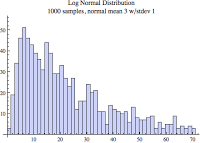Now that's more like it! Notice the far three left bars are not the tallest and also notice how far the tail extends to the right. These are two key identifying characteristics of the log normal distribution. But I have to warn you, there are many log normal distribution sample sets that do not have the far left bars less then the tallest bar. (e.g., country GDP data.) The only way to really test if your data is log normal is to perform a hypothesis test. I will detail this in the next blog entry.

### Predicting the median and mean

This is pretty cool: The actual data samples in the above histogram have an average of 53 and a median of 19. So there are enough large value samples to effectively pull the mean away from the median. If you recall, for both the normal and poisson distributions, the mean and median are equal.

Ready to be freaked out? For a log normal distribution, the median of its samples is supposed to be the constant e to the power of its normal distribution's average. If you recall above, the log normal sample set was created from normally distribution samples with a mean of 3. Therefore, the median equation is:

``````
median = e^m = e^3 = 2.718282846^3 = 20.08 ``````

Woah! Just above I said the actual median was 19, which is very close considering the sample set consists of only 1000 samples.

Let's try another freak-out thing: the mean of a log normal distribution is the constant e to the power of its normal distribution's average plus its standard deviation squared divided by two. Words are messy, so the equation is:

``````
mean = e^(m+(s^2)/2) = 2.718282846^(3+(1.5^2)/2) = 61.87 ``````

Again, not perfect but close...although I would have liked it to be closer. I will delve into the application of this in subsequent blog postings.

### Shifting sand

I mentioned above the input parameters to create a log normal distribution are the mean and standard deviation of its associated normal distribution. These two parameters are also sometimes referred to as the scale and shape parameters. If I mess with the shape parameter (i.e., standard deviation), this causes the histogram tail to either contract or extend far to the right. The scale parameter shifts the tallest histogram bar to the left or right.

Here's an example. In the image below the darkest red-ish color is the overlap of two histograms. The two histograms are colored purple and pink. The two data sets are only different in their scale parameter. You can see one of the differences is the tallest bar shifted to the right when the scale parameter was increased. Perhaps not that interesting, but it will be useful in future blog posts.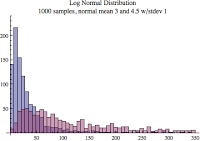The log normal distribution is amazing. The reason I focused so much on it is because it is important for Oracle performance analysis. I haven't demonstrated this yet, but I will in subsequent posts...stay tuned.

### Conclusions

As I dig deeper into Oracle performance analysis, I am forced to understand statistical distributions. There is just no way around it. Documenting my research and to prepare me to analyze experimental data has produced the content for this posting. My hope is that I have conveyed a few key take-aways:

A clear understanding of a histogram.

y changing histogram characteristics (e.g., bin size, number of bins) you can make a sample set look like a desired distribution.

How common statistical distributions relate to our lives.

What common statistical distribution histograms look like.

In subsequent blog postings, I will analyze how various Oracle system happenings relate to these common statistical distributions. This will allow us to communicate more confidently, more correctly, and also perhaps make some interesting predictions.